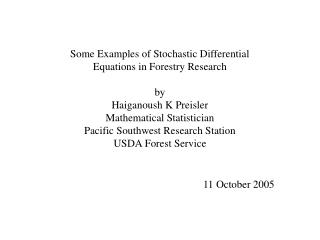DownloadDownload PresentationSome Examples of Stochastic Differential Equations in Forestry Research by Haiganoush K Preisler

# Some Examples of Stochastic Differential Equations in Forestry Research by Haiganoush K Preisler

Télécharger la présentation## Some Examples of Stochastic Differential Equations in Forestry Research by Haiganoush K Preisler

- - - - - - - - - - - - - - - - - - - - - - - - - - - E N D - - - - - - - - - - - - - - - - - - - - - - - - - - -
##### Presentation Transcript

1. Some Examples of Stochastic Differential Equations in Forestry Research by Haiganoush K Preisler Mathematical Statistician Pacific Southwest Research Station USDA Forest Service 11 October 2005

2. Black-Scholes formula 1997 Nobel Prize in Economic Sciences S(t) is value of a stock at time t B(t) is a Brownian process (random noise) Stochastic differential equation

3. Heat Equation Joseph Fourier (1768-1830) French Mathematician H(x,t)= temperature at depth x and time t.

4. Fourier used heat equation to predict temperature of the ground at depth x due to the sun’s heating. • Concluded • At certain depths temperatures are out of step with surface temperature (phase lag). • Six month lag at 2-3 m depth. • (i.e., hotter in winter, cooler in summer). • Good depth for cellars. • Permafros

5. Risks • Increase in mortality rates observed in soil organisms at 34 - 40o C. • Tissue cannot survive temperatures greater than 45 - 65o C.

6. Temperature Profiles at 10cm Depth Around Sugar Pine Trees 140 120 100 Temperature (Celsius) 80 60 40 20 0 0 20 40 60 80 100 120 Time (hrs) Temperature Profiles at 10 cm Depth Around Sequoia Trees 140 120 100 Temperature (Celsius) 80 60 40 20 0 0 20 40 60 80 100 120 Time (hrs) Different curves due to different soil conditions, some of which (e.g. soil moisture) are measured.

7. Rocky Mountain elk (Cervus elaphus)

8. a b 14 14 12 12 10 10 8 8 Km Km 6 6 4 4 2 2 0 0 0 2 4 6 8 10 0 2 4 6 8 10 Km Km Fenced area of Starkey Experimental Station in Oregon Tracks of four elk

9. Stochastic differential equations Differential equations r(t) = { x(t), y(t) } = location of elk at time t.

10. References • Preisler, H.K., Haase, A.M. and Sackett, S.S. (2000). Modeling and risk assessment for soil temperatures beneath prescribed forest fires. Environmental and Ecological Statistics 7, 239-254. • 2. Preisler, H.K., Ager, A.A., Johnson, B.K., and Kie, J.G. (2004) Modeling animal movements using stochastic differential equations. Environmetrics 15: 643-657.